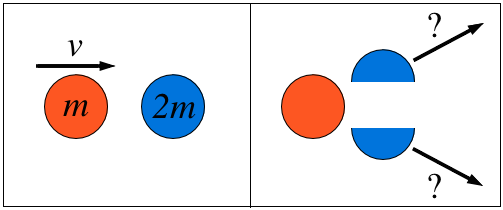# Collide and break!A body of mass $m$ is moving along the $x$-axis with speed $v$ when it collides with a body of mass $2m$ initially at rest. After the collision, the first body comes to rest and the second body splits into two pieces of equal mass that move with equal velocities at equal angles off the $x$-axis $0 < \theta < 90^\circ$, as shown in the figure.

Which of the following statements correctly describes the speeds of the two pieces?

×

Problem Loading...

Note Loading...

Set Loading...